Name:    Quiz 9.1-9.3

Multiple Choice
Identify the choice that best completes the statement or answers the question.

List the ordered pairs shown in the mapping diagram.

1.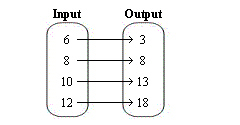a. (6, 3), (8, 8), (10, 13), (12, 18) c. (3, 12), (8, 10), (13, 8), (18, 6) b. (6, 8), (8, 13), (10, 18), (12, 3) d. (3, 6), (8, 8), (13, 10), (18, 12)

Find the value of y for the given value of x.

2.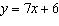;   x = 4.5
 a. 31.5 c. 10.5 b. 13 d. 37.5

3.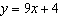;   x = 7
 a. 63 c. 67 b. 13 d. 11

Write an equation that describes the function.

4.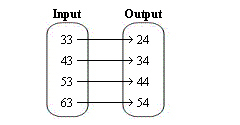a.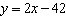c.b.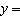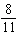x d.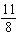x

5.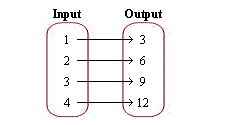a.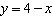c.6x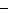3 b.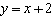d.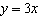6.

The output is eleven less than the input.
 a. y = 11 c.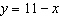b. y =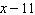d. y = 11x

7.

The output is two times the input.
 a.= 2y c.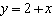b. y =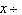2 d. y = 2x

Find the value of x for the given value of y.

8.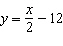;   y = 22
 a. 68 c. 56 b. 23 d. 20

Write an equation for the function shown by the table.

9.

 Input, x 7 8 9 10 Output, y 1 2 3 4
 a.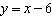c.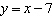b.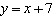d.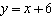10.

 Input, x 1 3 5 7 Output, y 12 36 60 84
 a.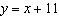c.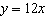b.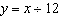d.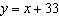Numeric Response

Find the value of y for the given value of x.

1.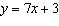;   x = 1

Write an equation for the function. Then complete the table.

1.

The output is 10 more than the input.
 Input, x 2 5 8 11 Output, y

2.

The output is 5 less than the input.
 Input, x 5 8 11 14 Output, y